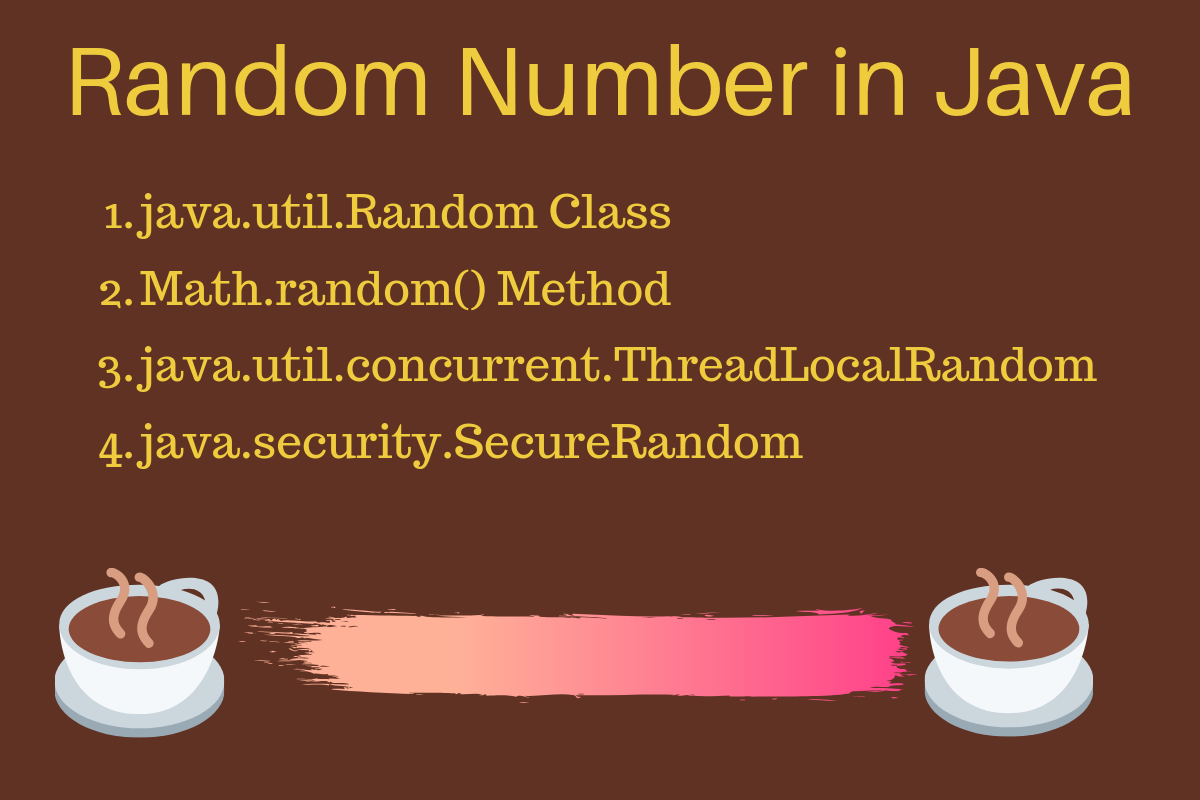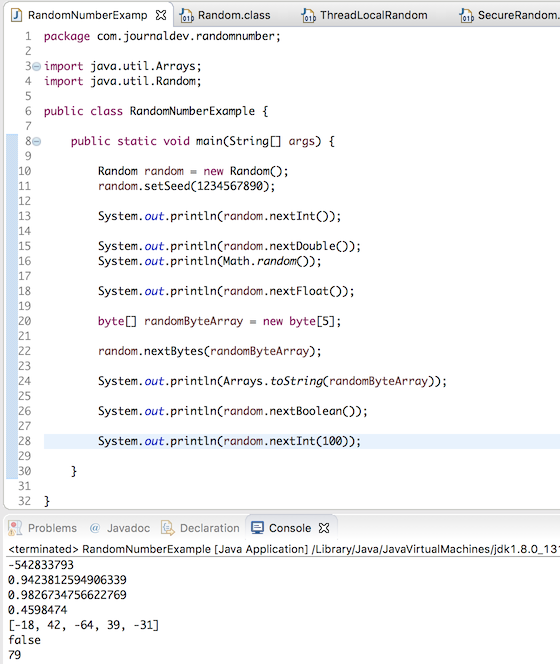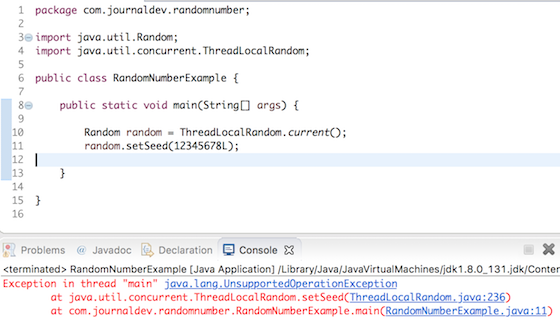# Random Number Generator in Java

Published on August 3, 2022By Pankaj
Developer and author at DigitalOcean.While we believe that this content benefits our community, we have not yet thoroughly reviewed it. If you have any suggestions for improvements, please let us know by clicking the “report an issue“ button at the bottom of the tutorial.

Today we will look at how to generate a random number in Java. Sometimes we need to generate random numbers in Java programs. For example, a dice game or to generate a random key id for encryption, etc.

## Random Number Generator in JavaThere are many ways to generate a random number in java.

1. java.util.Random class can be used to create random numbers. It provides several methods to generate random integer, long, double etc.
2. We can also use Math.random() to generate a double. This method internally uses Java Random class.
3. `java.util.concurrent.ThreadLocalRandom` class should be used to generate random number in multithreaded environment. This class is part of Java Concurrent package and introduced in Java 1.7. This class has methods similar to Java Random class.
4. `java.security.SecureRandom` can be used to generate random number with strong security. This class provides a cryptographically strong random number generator. However, it’s slow in processing. So depending on your application requirements, you should decide whether to use it or not.

## Java Random Number Generator

Let’s look at some examples to generate a random number in Java. Later on, we will also look at ThreadLocalRandom and SecureRandom example program.

### 1. Generate Random integer

``````Random random = new Random();

int rand = random.nextInt();
``````

Yes, it’s that simple to generate a random integer in java. When we create the Random instance, it generates a long seed value that is used in all the `nextXXX` method calls. We can set this seed value in the program, however, it’s not required in most of the cases.

``````//set the long seed value using Random constructor
Random random = new Random(123456789);

//set long seed value using setter method
Random random1 = new Random();
random1.setSeed(1234567890);
``````

### 2. Java Random number between 1 and 10

Sometimes we have to generate a random number between a range. For example, in a dice game possible values can be between 1 to 6 only. Below is the code showing how to generate a random number between 1 and 10 inclusive.

``````Random random = new Random();
int rand = 0;
while (true){
rand = random.nextInt(11);
if(rand !=0) break;
}
System.out.println(rand);
``````

The argument in the `nextInt(int x)` is excluded, so we have to provide argument as 11. Also, 0 is included in the generated random number, so we have to keep calling nextInt method until we get a value between 1 and 10. You can extend the above code to generate the random number within any given range.

### 3. Generate Random double

We can use `Math.random()` or Random class `nextDouble` method to generate random double number in java.

``````Random random = new Random();

double d = random.nextDouble();

double d1 = Math.random();
``````

### 4. Generate Random float

``````Random random = new Random();

float f = random.nextFloat();
``````

### 5. Generate Random long

``````Random random = new Random();

long l = random.nextLong();
``````

### 6. Generate Random boolean

``````Random random = new Random();

boolean flag = random.nextBoolean();
``````

### 7. Generate Random byte array

We can generate random bytes and place them into a user-supplied byte array using Random class. The number of random bytes produced is equal to the length of the byte array.

``````Random random = new Random();

byte[] randomByteArray = new byte;

random.nextBytes(randomByteArray);

System.out.println(Arrays.toString(randomByteArray)); // sample output [-70, -57, 74, 99, -78]
``````

Here is a simple example showing ThreadLocalRandom usage in a multithreaded environment.

``````package com.journaldev.randomnumber;

import java.util.Random;

public class ThreadLocalRandomExample {

public static void main(String[] args) {
Runnable runnable = new MyRunnable();

for (int i = 0; i < 5; i++) {
t.start();
}
}

}

class MyRunnable implements Runnable {

@Override
public void run() {
}

}
``````

Below is a sample output of my execution of the above program.

``````MyRunnable-Thread-0::-1744088963
``````

We can’t set seed value for ThreadLocalRandom instance, it will throw `UnsupportedOperationException`.ThreadLocalRandom class also has some extra utility methods to generate a random number within a range. For example, to generate a random number between 1 and 10, we can do it like below.

``````ThreadLocalRandom random = ThreadLocalRandom.current();

int rand = random.nextInt(1, 11);
``````

ThreadLocalRandom has similar methods for generating random long and double values.

### 9. SecureRandom Example

You can use SecureRandom class to generate more secure random numbers using any of the listed providers. A quick SecureRandom example code is given below.

``````Random random = new SecureRandom();

int rand = random.nextInt();

System.out.println(rand);
``````

That’s all about generating a random number in Java program.

You can download the example code from our GitHub Repository.

### Want to learn more? Join the DigitalOcean Community!

Join our DigitalOcean community of over a million developers for free! Get help and share knowledge in our Questions & Answers section, find tutorials and tools that will help you grow as a developer and scale your project or business, and subscribe to topics of interest.Pankaj

author

Developer and author at DigitalOcean.